Next: Converted feedforward equations Up: Computing the expectation of Previous: Expectation of a function

## Variance of a function

We still need the variances vj. Since each error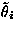is evenly distributed in the range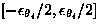, the variances of the parameters are given by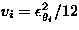. The variance of the inputs and desired outputs can be assumed to be zero, or they can be assigned some values if there is prior knowledge about, for example, the equipment used to measure the values.

In order to compute the variance of the function fi, we shall approximate it with first order Taylor's series expansion.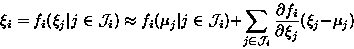(7)
According to this approximation,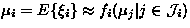, which yields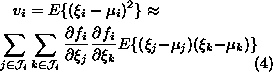Again we can drop out the cross terms if all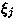are mutually uncorrelated. This yields our final approximation for the variance of a function: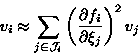(8)
Notice that when computing the variance, one cannot mix the first and second order approximation for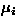, since that might result in negative values for vi.

Harri Lappalainen
5/19/1998